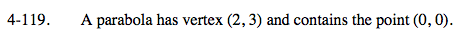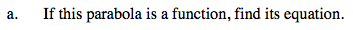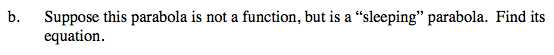### Home > A2C > Chapter 4 > Lesson 4.2.4 > Problem4-119

4-119.Use the equation y = a(xh)2 + k; if (h, k) = (2, 3); at (0, 0).

0 = a(0 − 2)2 + 3

−3 = a(4)

$-\frac{3}{4}=\textit{a}$

$\textit{y}=-\frac{3}{4}(\textit{x}-2)^{2}+3$Use the equation x = b(yk)2 + h; if (h, k) = (2, 3); at (0, 0).

Use the eTool below to graph the equations.
Click the link at right for the full version of the eTool: A2C 4-119 HW eTool